# Some Basic Math Creates A Low-Cost Nonlinear Thermocouple Interface

## Analog Devices AD633 LT1025 OP177GS

Measurement and process control loops often use 8-bit microcontrollers. The devices are inexpensive and widely available, and they can be programmed in many popular high-level languages – like C and Basic. However, if the loop requires a nonlinear sensor, the designer faces the added challenge of having to develop a software linearization algorithm.

One solution is to design hardware to perform the required curve fitting before the sensor’s output signal is applied to the microcontroller’s analog-to-digital converter (ADC) input. This is an especially attractive solution if processor memory is limited and cost and component count can be kept low. An added benefit is a small printed-circuit board (PCB) footprint.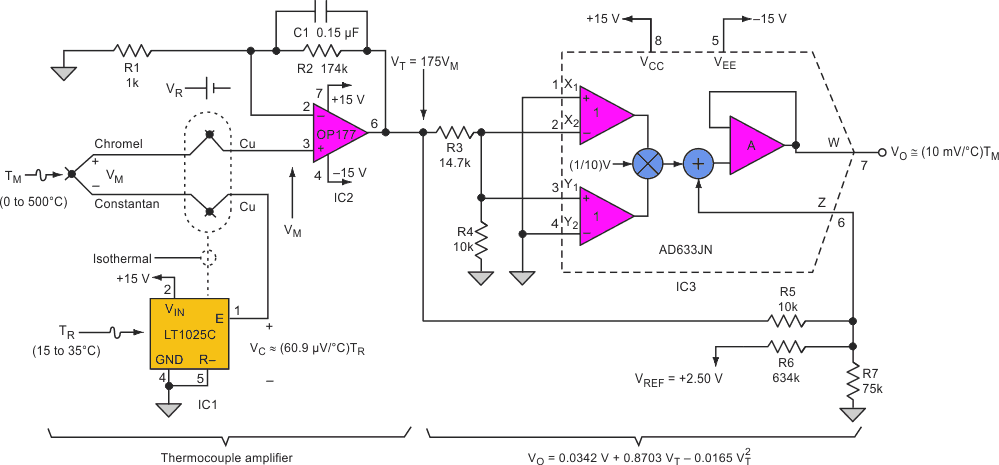Figure 1. Instead of a software linearization algorithm, this circuit uses a hardware solution to perform the required curve fitting for a nonlinear sensor.

An example is the circuit shown in the Figure 1, which uses a type-E thermocouple to measure temperatures that are expected to vary from 0 °C to 500 °C. The circuit’s output ranges from 0 to 5 V – the full span of the ADC – and can be expressed as a linear system equation: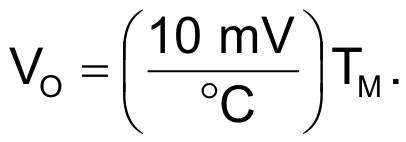(1)

The thermocouple amplifier section consists of a thermocouple cold-junction compensator (IC1), an op amp (IC2), and accompanying circuitry. IC1 tracks ambient temperature (TR) and adds a correction voltage (VC) to cancel the cold-junction voltage (VR) created when chromel-constantan thermocouple leads are attached to the copper pads of a PCB. IC2 then amplifies the thermocouple’s temperature-dependent signal (VM) by 175 to produce VT prior to linearization. A gain of 175 eliminates the need for additional amplification during curve fitting.

Low-pass filter C1-R2 has a pole at approximately 6 Hz to remove power-supply noise.

Table 1. The temperature entries for TM with their
corresponding thermoelectric voltages
 TM (°C) VM (V) VT = 175 VM (V) VO (V) 0 0 0 0 50 0.003047 0.533225 0.5 100 0.006317 1.105475 1 150 0.009787 1.712725 1.5 200 0.013419 2.348325 2 250 0.017178 3.00615 2.5 300 0.021033 3.680775 3 350 0.024961 4.368175 3.5 400 0.028943 5.065025 4 450 0.03296 5.768 4.5 500 0.036999 6.474825 5

You can use an Excel spreadsheet to create the nonlinear mathematical relationship between the output of the thermocouple amplifier (VT) and the input to the ADC (VO). The Table 1 shows 11 temperature entries for TM (0 °C to 500 °C in 50 °C steps) with their corresponding thermoelectric voltages, VM. The values of VM were derived from a standard type-E thermocouple reference table. Also shown are VT and VO, which are charted using the (XY) Scatter feature of the software. The equation for VO is created using the software’s trendline feature: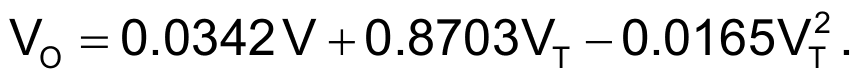(2)

An analog multiplier (IC3) and five 1% resistors implement this equation, a second-order polynomial of the form: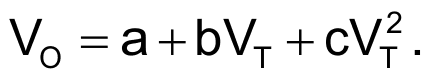(3)

The four (X and Y) inputs of IC3 are wired to create a negative square term that’s scaled at the chip’s output by an internal scale factor of 0.1 V. Comparing terms, we note that c must equal 0.0165. Since R3 and R4 form a voltage divider to attenuate VT, we can express c as follows: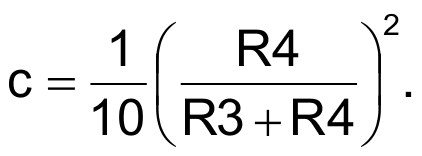(4)

You can then solve for R3 by substituting c = 0.0165 and selecting a value for R4, which for this design was chosen to be 10 kΩ.

Resistors R5, R6, and R7 form a passive adder to create the offset term, a, and the linear coefficient, b, in Equation 3. The output of the passive adder is applied directly to the Z-input of IC3, which adds the offset and linear terms to the square term. Again comparing terms, a (Equation 3) equals 0.0342 V (Equation 2). We can express this as: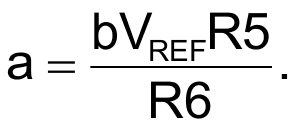(5)

To design this part of the passive adder, we chose a stable 2.500-V reference for VREF, selected R5 to be 10 kΩ, and solved for R6.

Finally, compare b (Equation 3) with the corresponding value of 0.8703 (Equation 2) and express the linear coefficient b as: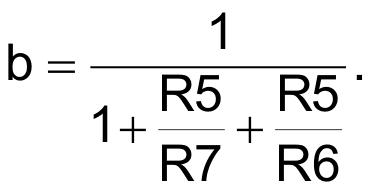(6)

This equation is solved to determine the final component, R7: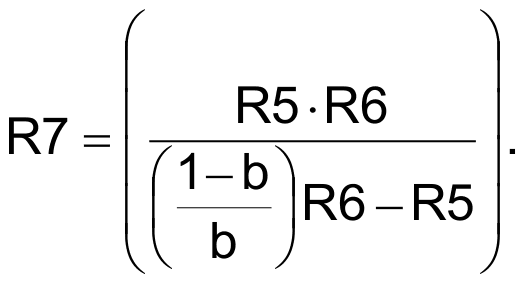(7)

We evaluated the circuit by replacing the thermocouple with a low-impedance voltage source to simulate VM. IC3’s output voltage (VO) exhibited a worst-case error of about 3.4 °C at 0 °C (VO = 0.034 V) and an error of –1.8 °C at 500 °C (VO = 4.982 V) at full scale. At 250 °C, a mid-range error of 0.2 °C (VO = 2.502 V) was recorded.

## Reference

1. IC Generates Second-Order Polynomial,” Electronic Design, Aug. 5, 1993, p . 83.

## Materials on the topic

electronicdesign.com